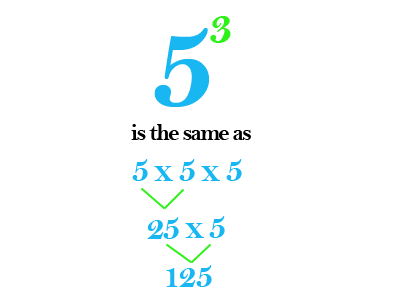Select Page

The Math Movie Network is back, bringing you the latest from our sixth grade classroom! This time, some of our experts provide you with a look at how to solve problems with exponents and the order of operations.

One of the topics that we’ve been learning all about are exponents. Much like where multiplication is used instead of repeated addition, exponents are used in lieu of repeated multiplication.Take a look as Ryan, Taite, Sam, and Bryan share how to solve exponents!

We’ve also been learning about the order of operations. Sometimes, you’ll find a math problem that contains more than one operation in it. When this occurs, there is a certain order in which the problem must be solved. We often use the term PEMDAS to help remind us of the order. PEMDAS stands for parentheses, exponents, multiplication, division, addition, and subtraction.

Kylie, Rachele, Tessa, and Allison will share a couple examples of problems in which the order of operations is used.

Thanks for watching!

*What are some examples times where you might have to use an exponent?*

*When might you need to use more than one operation in a problem?*

Subscribe By Email

Get a weekly email of all new posts.